## 3.5 The Question of Compatibility

We now have two routes to thetheory. In Section 3.2 we saw how to reach it via thetheory:Our work in that section and in Section 3.1 showed that this diagram commutes, which is a way of saying that thetheory extends the Standard Model.

In Section 3.4 we saw another route to thetheory, which goes through:Our work in that section and Section 3.3 showed that this diagram commutes as well. So, we have another way to extend the Standard Model and get thetheory.

Drawing these two routes totogether gives us a cube: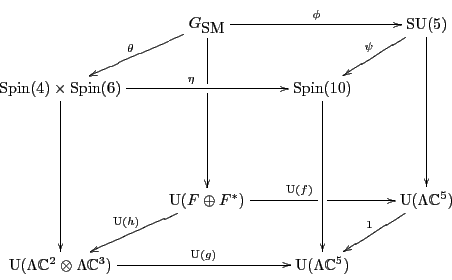Are these two routes totheory the same? That is, does the cube commute?

Theorem 7   . The cube commutes.

Proof. We have already seen in Sections 3.1-3.4 that the vertical faces commute. So, we are left with two questions involving the horizontal faces. First: does the top face of the cubecommute? In other words: does a symmetry in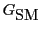go to the same place inno matter how we take it there? And second: does the bottom face of the cube commute? In other words: does this triangle:commute?

In fact they both do, and we can use our affirmative answer to the second question to settle the first. As we remarked in Section 3.4, applying the map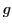to the Pati-Salam binary code given in Table 6, we get thebinary code given in Table 4. Thus, the linear mapsandagree on a basis, so this triangle commutes:This in turn implies that the bottom face of the cube commutes, from which we see that the two maps fromto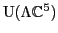going around the bottom face are equal:The work of Section 3.1 through Section 3.4 showed that the vertical faces of the cube commute. We can thus conclude from diagrammatic reasoning that the two maps fromtogoing around the top face are equal:Since the Dirac spinor representation is faithful, the map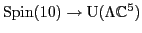is injective. This means we can drop it from the above diagram, and the remaining square commutes. But this is exactly the top face of the cube. So, the proof is done.2010-01-11